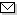Language:   Search:   ContactSearch and Browse the MSC 2000

Search:
Please note that in the second half of 2009, MSC2010 is deployed in the production of ZMATH and will become official standard in January 2010.

Enter a query and click »Search«...

58-XX Global analysis, analysis on manifolds {For geometric integration theory, see 49Q15} → ZMATH
58Jxx Partial differential equations on manifolds; differential operators [See also 32Wxx, 35-XX, 53Cxx] → ZMATH
58J05 Elliptic equations on manifolds, general theory [See also 35-XX] → ZMATH
58J10 Differential complexes [See also 35Nxx]; elliptic complexes → ZMATH
58J15 Relations with hyperfunctions → ZMATH
58J20 Index theory and related fixed point theorems [See also 19K56, 46L80] → ZMATH
58J22 Exotic index theories [See also 19K56, 46L05, 46L10, 46L80, 46M20] → ZMATH
58J26 Elliptic genera → ZMATH
58J28 Eta-invariants, Chern-Simons invariants → ZMATH
58J30 Spectral flows → ZMATH
58J32 Boundary value problems on manifolds → ZMATH
58J35 Heat and other parabolic equation methods → ZMATH
58J37 Perturbations; asymptotics → ZMATH
58J40 Pseudodifferential and Fourier integral operators on manifolds [See also 35Sxx] → ZMATH
58J42 Noncommutative global analysis, noncommutative residues → ZMATH
58J45 Hyperbolic equations [See also 35Lxx] → ZMATH
58J47 Propagation of singularities; initial value problems → ZMATH
58J50 Spectral problems; spectral geometry; scattering theory [See also 35Pxx] → ZMATH
58J52 Determinants and determinant bundles, analytic torsion → ZMATH
58J53 Isospectrality → ZMATH
58J55 Bifurcation [See also 35B32] → ZMATH
58J60 Relations with special manifold structures (Riemannian, Finsler, etc.) → ZMATH
58J65 Diffusion processes and stochastic analysis on manifolds [See also 35R60, 60H10, 60J60] → ZMATH
58J70 Invariance and symmetry properties [See also 35A30] → ZMATH
58J72 Correspondences and other transformation methods (e.g. Lie-Bäcklund) [See also 35A22] → ZMATH
58J90 Applications → ZMATH
58J99 None of the above, but in this section → ZMATH

NewsAbel prize 2010I. M. Gelfand 1913-2009MSC2010

Copyright © 2019 Zentralblatt MATH | European Mathematical Society | FIZ Karlsruhe | Heidelberg Academy of Sciences